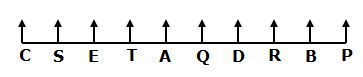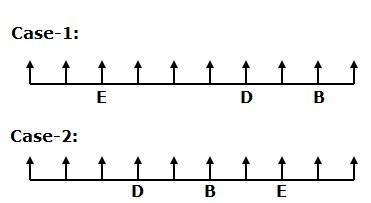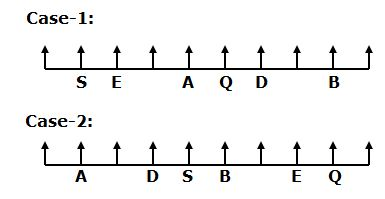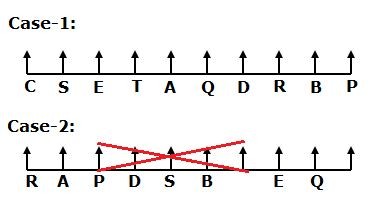# CWC/FCI Prelims 2019 Reasoning Ability Questions (Day-10)

Dear Readers, Exam Race for the Year 2019 has already started, To enrich your preparation here we are providing new series of Practice Questions on Reasoning Ability– Section for CWC/FCI Exam. Aspirants, practice these questions on a regular basis to improve your score in aptitude section. Start your effective preparation from the right beginning to get success in upcoming CWC/FCI Exam.

[WpProQuiz 5499]

Direction (1-5): Read the following information carefully and answer the questions given below.

Ten persons A, B, C, D, E, P, Q, R, S and T are sitting in a straight row with equidistance from each other. They all are facing north but not necessary in the same order.

P sits second to the right of R. Only three persons sitting between E and D. B sits second to the right of D. Q sits fourth to the right of S. As many persons sitting to the left of A is same as the persons sitting to the right of Q. As many persons sitting between A and R is same as the persons sitting between D and T. E sits at third from the either ends of the row. Neither S nor Q sits at extreme ends of the row.

1) Who among the following sits second to the right of C?

a) T

b) S

c) Q

d) E

e) None of these

2) How many persons are sitting between R and A?

a) One

b) Two

c) Three

d) None

e) More than three

3) If S is related to B and D is related to T in a certain way. Then, E is related to which of the following?

a) C

b) P

c) Q

d) R

e) None of these

4) Four of the following five are alike in a certain way and hence form a group. Which one of the following that does not belong to the group?

a) QR

b) EA

c) TC

d) DB

e) ST

5) Which of the following statements is true?

a) More than three persons sitting between Q and P

b) P sits at one of the extreme ends of the row

c) T sits second to the right of Q

d) E and C are immediate neighbours

e) None is true

Directions (6-10): In these questions relationship between different elements is shown in the statements. These statements are followed by conclusions. You have to find out which of the conclusions follow or not from the given statements. Give answer,

(a) If only conclusion I is true

(b) If only conclusion II is true

(c) If either conclusions I or II is true

(d) If neither conclusion I nor II is true

(e) If both conclusions I and II are true

6) Statement: G<V≤D=A>R; H≥R≥W<U≤P.

Conclusions:

I. P≥V

II. W<D

7) Statement: N=H>D≥X≥Y; L>K=S≥D≤R.

Conclusions:

I. Y≤K

II. L>X

8) Statement: J≤H=Z<D≥T; M≥L>P=H≤Q.

Conclusions:

D>L

J≤M

9) Statement: C≥S>U=W≥Z; V<U≤F=P>M.

Conclusions:

I. Z<P

II. F=Z

10) Statement: B<V=C≤S≤R; S≤T≤L>P=A.

Conclusions:

I. C<P

II. A≤R

Direction (1-5):• Only three persons sitting between E and D. E sits at third from the either ends of the row. B sits second to the right of D.• Q sits fourth to the right of S. As many persons sitting to the left of A is same as the persons sitting to the right of Q. Neither S nor Q sits at extreme ends of the row.• As many persons sitting between A and R is same as the persons sitting between D and T. P sits second to the right of R.
• So, Case-2 will be dropped.Directions (6-10):

I. P≥V –> V≤D=A>R≥W<U≤P

II. W<D –> W≤R<A=D

I. Y≤K –> Y≤X≤D≤S=K

II. L>X –> L>K=S≥D≥X

I. D>L –> D>Z=H=P<L

II. J≤M –> J≤H=P<L≤M

I. Z<P –> Z≤W=U≤F=P

II. F=Z –> Z≤W=U≤F